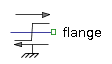2-port Demultiplexer - MapleSim Help

2-port Demultiplexer

Separates a real input signal into two output signalsImportant: The 2-port Demultiplexer component has been deprecated. Instead, use the   n-port Boolean Demultiplexer to separate a boolean input signal into output signals,  the   n-port Integer Demultiplexer to separate an integer input signal into output signals or the n-port Real Demultiplexer to separate a real input signal into output signals.  The number of output ports can be set by the dimension of the parameter between 2 and 10.

 Description The 2-port Demultiplexer component separates the input signal, $u$, into two output signals, ${y}_{1}$and ${y}_{2}$, in the specified dimensions.

Connections

 Name Description $u$ Real input signal ${y}_{1}$ Real output signal ${y}_{2}$ Real output signal

Variables

 Symbol Units Description $u$ - Physical signal at the input port $y$ - Physical signal at the output port

Parameters

 Symbol Default Units Description Modelica ID ${n}_{1}$ $1$ - Dimension of output signal connector 1 n1 ${n}_{2}$ $1$ - Dimension of output signal connector 2 n2